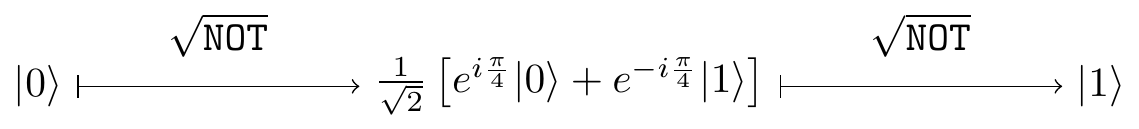## 2.12Remarks and exercises

### 2.12.1 Unknown phase

Consider the usual quantum interference circuit:Suppose you can control the input of the circuit and measure the output, but you do not know the phase shift \varphi introduced by the phase gate. You prepare input |0\rangle and register output |1\rangle. What can you say about \varphi?

Now you are promised that \varphi is either 0 or \pi. You can run the circuit only once to find out which of the two phases was chosen. Is it possible to then always correctly guess whether \varphi was 0 or \pi?

### 2.12.2 One of the many cross-product identities

When working with three-dimensional geometry, the cross product of vectors is very useful, so here is an exercise to help you get used to working with it. Derive the identity (\vec{a}\cdot\vec{\sigma})(\vec{b}\cdot\vec{\sigma}) = (\vec{a}\cdot\vec{b})\mathbf{1}+ i(\vec{a}\times \vec{b})\cdot \vec{\sigma}.

Hint: all you need here are the Pauli matrices’ commutation and anti-commutation relations, but it is instructive to derive the identity using the component notation, and below we give a sketch of how such a derivation would go.

First, notice that the products of Pauli matrices can be written succinctly as \sigma_{i}\sigma_{j} = \delta _{ij}\mathbf{1}+ i\varepsilon_{ijk}\,\sigma _{k}, where \delta_{ij} is Kronecker delta (equal to 0 if i\neq j, and to 1 if i=j) and \varepsilon_{ijk} is the Levi-Civita symbol: \varepsilon_{ijk} = \begin{cases} +1 & {\text{if }}(i,j,k){\text{ is }}(1,2,3)\text{, }(2,3,1){\text{, or }}(3,1,2) \\-1 & {\text{if }}(i,j,k){\text{ is }}(3,2,1)\text{, }(1,3,2){\text{, or }}(2,1,3) \\\;\;\;0 & {\text{if }}i=j,{\text{ or }}j=k,{\text{ or }}k=i \end{cases} That is, \varepsilon _{ijk} is 1 if (i, j, k) is an even permutation of (1, 2, 3), it is -1 if it is an odd permutation, and it is 0 if any index is repeated. The Levi-Civita symbol is anti-symmetric, meaning when any two indices are changed, its sign alternates. Then recall that the scalar (dot) product and vector (cross) product of two Euclidean vectors \vec{a} and \vec{b} can be written, in terms of the components, as \begin{aligned} \vec{a}\cdot\vec{b} &= \sum_{i=1}^3 a_i b_i \\(\vec{a}\times\vec{b})_i &= \sum_{j,k=1}^3 \varepsilon_{ijk}a_jb_k. \end{aligned} The rest is rather straightforward: (\vec{a}\cdot\vec{\sigma})(\vec{b}\cdot\vec{\sigma}) = \sum_{ij}a_i b_j\sigma_i\sigma_j = \ldots.